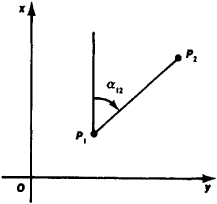# Direction Angle

## Direction Angle

an angle that characterizes the direction of an arbitrary straight line with respect to an initial direction, particularly with respect to a coordinate axis. In geodesy, a distinction is made between a direction angle on an ellipsoid and a direction angle in a plane (of a projection or of a topographical map). In a plane (Figure 1), the direction angle α12, which at the point P1 has the direction P1P2 to the point P2, is defined as the angle at P1 (in a clockwise direction) between the straight line parallel to the .Y-axis and the directed line P1P2. The direction angle is a basic concept in geodesy and is also used in artillery, navigation, and other fields.Figure 1

References in periodicals archive ?
In this first case, a sinusoidal speed and direction angle references were used to test the effectiveness of the PI-based MRAS and the proposed FO-MRAS controller.
The thrust direction angle [gamma] is the intersection angle from [F.sub.[alpha]] to F.
Figure 6 shows the illustration of FDA antenna's apparent direction angle and physical direction angle.
The range of simulations allows consideration of "under-merged" plumes with low plume cone angle and high plume direction angle, or "over-merged" plumes with high plume cone angle and low plume direction angle.
The starting point of the path is (0, 0), and direction angle is 0[degrees].
Base shear, bending moment, and the acceleration response are calculated as function of the direction angle [alpha], for several damping ratios [xi] (1.0%; 2.0%; and 4.0%) (Figures 7 and 8).
Let a be a point in R2 , and n(8) = ( cos(8), sin(8)) be a unit vector in R2 , where 8 is direction angle. We consider the partition of R2 given by
The proposed method estimates positioning accuracy based on direction angle, velocity, and distance using an accurate reference point and long-term averaging, rounding error reduction via coordinate translation, and an invalid data check.
The average bistatic NRCS results versus scattering angles from linear, CWM and Creamer (2) sea surfaces for indent angles of 0[degrees] and 60[degrees] in wind direction angle of 45[degrees] are shown in Figures 5(a), (b) (wind speed of 3 m/s).
The principal damage values [D.sub.1] and [D.sub.2] for fracture sample are 0.17 for minimum and 0.50 for maximum, and the principal direction angle [theta] of damage tensor is approximately 105[degrees] which is perpendicular to the direction of principal permeability.
Two six-port networks are used to determine the beam direction angle. Figure 1 shows the block schematic of a direction finding receiver.

Site: Follow: Share:
Open / Close